GeeksforGeeks App
Open AppBrowser
Continue

# Selecting rows in pandas DataFrame based on conditions

Let’s see how to Select rows based on some conditions in Pandas DataFrame.

### Selecting rows based on particular column value using `'>', '=', '=', '<=', '!=' `operator.

Code #1 : Selecting all the rows from the given dataframe in which ‘Percentage’ is greater than 80 using basic method.

 `# importing pandas``import` `pandas as pd`` ` `record ``=` `{`` ` ` ``'Name'``: [``'Ankit'``, ``'Amit'``, ``'Aishwarya'``, ``'Priyanka'``, ``'Priya'``, ``'Shaurya'` `],`` ``'Age'``: [``21``, ``19``, ``20``, ``18``, ``17``, ``21``],`` ``'Stream'``: [``'Math'``, ``'Commerce'``, ``'Science'``, ``'Math'``, ``'Math'``, ``'Science'``],`` ``'Percentage'``: [``88``, ``92``, ``95``, ``70``, ``65``, ``78``] }`` ` `# create a dataframe``dataframe ``=` `pd.DataFrame(record, columns ``=` `[``'Name'``, ``'Age'``, ``'Stream'``, ``'Percentage'``])`` ` `print``(``"Given Dataframe :\n"``, dataframe) `` ` `# selecting rows based on condition``rslt_df ``=` `dataframe[dataframe[``'Percentage'``] > ``80``]`` ` `print``(``'\nResult dataframe :\n'``, rslt_df)`

Output :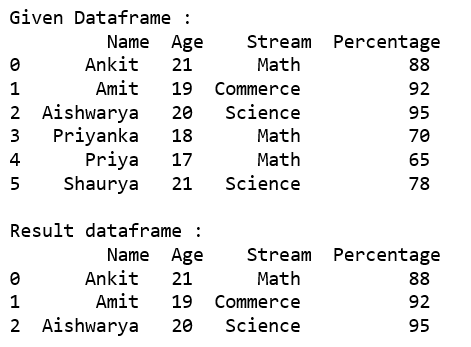Code #2 : Selecting all the rows from the given dataframe in which ‘Percentage’ is greater than 80 using `loc[]`.

 `# importing pandas``import` `pandas as pd`` ` `record ``=` `{``  ``'Name'``: [``'Ankit'``, ``'Amit'``, ``'Aishwarya'``, ``'Priyanka'``, ``'Priya'``, ``'Shaurya'` `],``  ``'Age'``: [``21``, ``19``, ``20``, ``18``, ``17``, ``21``],``  ``'Stream'``: [``'Math'``, ``'Commerce'``, ``'Science'``, ``'Math'``, ``'Math'``, ``'Science'``],``  ``'Percentage'``: [``88``, ``92``, ``95``, ``70``, ``65``, ``78``]}`` ` `# create a dataframe``dataframe ``=` `pd.DataFrame(record, columns ``=` `[``'Name'``, ``'Age'``, ``'Stream'``, ``'Percentage'``])`` ` `print``(``"Given Dataframe :\n"``, dataframe) `` ` `# selecting rows based on condition``rslt_df ``=` `dataframe.loc[dataframe[``'Percentage'``] > ``80``]`` ` `print``(``'\nResult dataframe :\n'``, rslt_df)`

Output :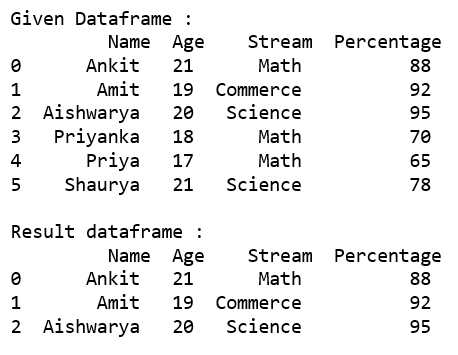Code #3 : Selecting all the rows from the given dataframe in which ‘Percentage’ is not equal to 95 using `loc[]`.

 `# importing pandas``import` `pandas as pd`` ` `record ``=` `{``  ``'Name'``: [``'Ankit'``, ``'Amit'``, ``'Aishwarya'``, ``'Priyanka'``, ``'Priya'``, ``'Shaurya'` `],``  ``'Age'``: [``21``, ``19``, ``20``, ``18``, ``17``, ``21``],``  ``'Stream'``: [``'Math'``, ``'Commerce'``, ``'Science'``, ``'Math'``, ``'Math'``, ``'Science'``],``  ``'Percentage'``: [``88``, ``92``, ``95``, ``70``, ``65``, ``78``]}`` ` `# create a dataframe``dataframe ``=` `pd.DataFrame(record, columns ``=` `[``'Name'``, ``'Age'``, ``'Stream'``, ``'Percentage'``])`` ` `print``(``"Given Dataframe :\n"``, dataframe) `` ` `# selecting rows based on condition``rslt_df ``=` `dataframe.loc[dataframe[``'Percentage'``] !``=` `95``]`` ` `print``(``'\nResult dataframe :\n'``, rslt_df)`

Output :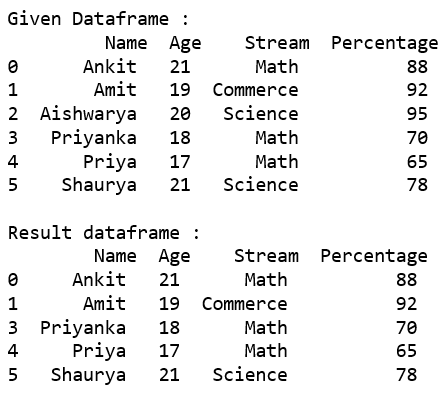### Selecting those rows whose column value is present in the list using `isin()` method of the dataframe.

Code #1 : Selecting all the rows from the given dataframe in which ‘Stream’ is present in the options list using basic method.

 `# importing pandas``import` `pandas as pd`` ` `record ``=` `{``  ``'Name'``: [``'Ankit'``, ``'Amit'``, ``'Aishwarya'``, ``'Priyanka'``, ``'Priya'``, ``'Shaurya'` `],``  ``'Age'``: [``21``, ``19``, ``20``, ``18``, ``17``, ``21``],``  ``'Stream'``: [``'Math'``, ``'Commerce'``, ``'Science'``, ``'Math'``, ``'Math'``, ``'Science'``],``  ``'Percentage'``: [``88``, ``92``, ``95``, ``70``, ``65``, ``78``]}`` ` `# create a dataframe``dataframe ``=` `pd.DataFrame(record, columns ``=` `[``'Name'``, ``'Age'``, ``'Stream'``, ``'Percentage'``])`` ` `print``(``"Given Dataframe :\n"``, dataframe) `` ` `options ``=` `[``'Math'``, ``'Commerce'``]`` ` `# selecting rows based on condition``rslt_df ``=` `dataframe[dataframe[``'Stream'``].isin(options)]`` ` `print``(``'\nResult dataframe :\n'``, rslt_df)`

Output :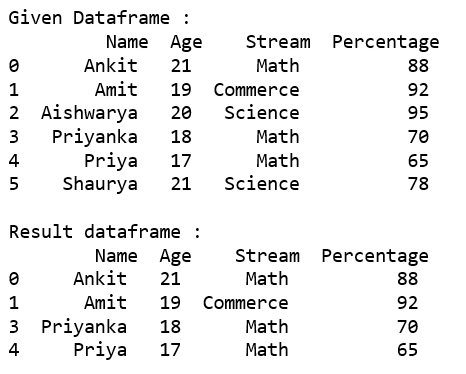Code #2 : Selecting all the rows from the given dataframe in which ‘Stream’ is present in the options list using `loc[]`.

 `# importing pandas``import` `pandas as pd`` ` `record ``=` `{``  ``'Name'``: [``'Ankit'``, ``'Amit'``, ``'Aishwarya'``, ``'Priyanka'``, ``'Priya'``, ``'Shaurya'` `],``  ``'Age'``: [``21``, ``19``, ``20``, ``18``, ``17``, ``21``],``  ``'Stream'``: [``'Math'``, ``'Commerce'``, ``'Science'``, ``'Math'``, ``'Math'``, ``'Science'``],``  ``'Percentage'``: [``88``, ``92``, ``95``, ``70``, ``65``, ``78``]}`` ` `# create a dataframe``dataframe ``=` `pd.DataFrame(record, columns ``=` `[``'Name'``, ``'Age'``, ``'Stream'``, ``'Percentage'``])`` ` `print``(``"Given Dataframe :\n"``, dataframe) `` ` `options ``=` `[``'Math'``, ``'Commerce'``]`` ` `# selecting rows based on condition``rslt_df ``=` `dataframe.loc[dataframe[``'Stream'``].isin(options)]`` ` `print``(``'\nResult dataframe :\n'``, rslt_df)`

Output :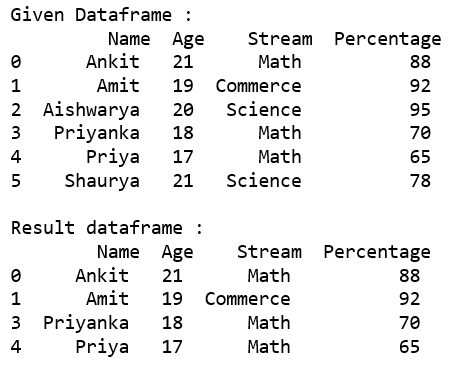Code #3 : Selecting all the rows from the given dataframe in which ‘Stream’ is not present in the options list using `.loc[]`.

 `# importing pandas``import` `pandas as pd`` ` `record ``=` `{``  ``'Name'``: [``'Ankit'``, ``'Amit'``, ``'Aishwarya'``, ``'Priyanka'``, ``'Priya'``, ``'Shaurya'` `],``  ``'Age'``: [``21``, ``19``, ``20``, ``18``, ``17``, ``21``],``  ``'Stream'``: [``'Math'``, ``'Commerce'``, ``'Science'``, ``'Math'``, ``'Math'``, ``'Science'``],``  ``'Percentage'``: [``88``, ``92``, ``95``, ``70``, ``65``, ``78``]}`` ` `# create a dataframe``dataframe ``=` `pd.DataFrame(record, columns ``=` `[``'Name'``, ``'Age'``, ``'Stream'``, ``'Percentage'``])`` ` `print``(``"Given Dataframe :\n"``, dataframe) `` ` `options ``=` `[``'Math'``, ``'Science'``]`` ` `# selecting rows based on condition``rslt_df ``=` `dataframe.loc[~dataframe[``'Stream'``].isin(options)]`` ` `print``(``'\nresult dataframe :\n'``, rslt_df)`

Output :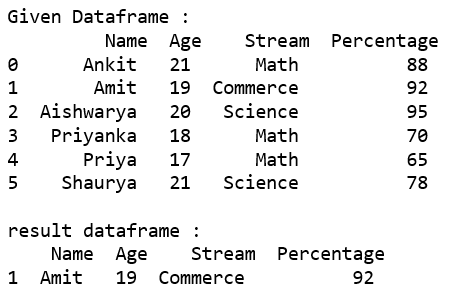### Selecting rows based on multiple column conditions using `'&'` operator.

Code #1 : Selecting all the rows from the given dataframe in which ‘Age’ is equal to 21 and ‘Stream’ is present in the options list using basic method.

 `# importing pandas``import` `pandas as pd`` ` `record ``=` `{``  ``'Name'``: [``'Ankit'``, ``'Amit'``, ``'Aishwarya'``, ``'Priyanka'``, ``'Priya'``, ``'Shaurya'` `],``  ``'Age'``: [``21``, ``19``, ``20``, ``18``, ``17``, ``21``],``  ``'Stream'``: [``'Math'``, ``'Commerce'``, ``'Science'``, ``'Math'``, ``'Math'``, ``'Science'``],``  ``'Percentage'``: [``88``, ``92``, ``95``, ``70``, ``65``, ``78``]}`` ` `# create a dataframe``dataframe ``=` `pd.DataFrame(record, columns ``=` `[``'Name'``, ``'Age'``, ``'Stream'``, ``'Percentage'``])`` ` `print``(``"Given Dataframe :\n"``, dataframe) `` ` `options ``=` `[``'Math'``, ``'Science'``]`` ` `# selecting rows based on condition``rslt_df ``=` `dataframe[(dataframe[``'Age'``] ``=``=` `21``) &``          ``dataframe[``'Stream'``].isin(options)]`` ` `print``(``'\nResult dataframe :\n'``, rslt_df)`

Output :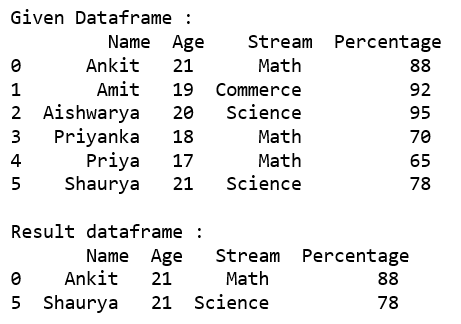Code #2 : Selecting all the rows from the given dataframe in which ‘Age’ is equal to 21 and ‘Stream’ is present in the options list using .loc[].

 `# importing pandas``import` `pandas as pd`` ` `record ``=` `{``  ``'Name'``: [``'Ankit'``, ``'Amit'``, ``'Aishwarya'``, ``'Priyanka'``, ``'Priya'``, ``'Shaurya'` `],``  ``'Age'``: [``21``, ``19``, ``20``, ``18``, ``17``, ``21``],``  ``'Stream'``: [``'Math'``, ``'Commerce'``, ``'Science'``, ``'Math'``, ``'Math'``, ``'Science'``],``  ``'Percentage'``: [``88``, ``92``, ``95``, ``70``, ``65``, ``78``]}`` ` `# create a dataframe``dataframe ``=` `pd.DataFrame(record, columns ``=` `[``'Name'``, ``'Age'``, ``'Stream'``, ``'Percentage'``])`` ` `print``(``"Given Dataframe :\n"``, dataframe) `` ` `options ``=` `[``'Math'``, ``'Science'``]`` ` `# selecting rows based on condition``rslt_df ``=` `dataframe.loc[(dataframe[``'Age'``] ``=``=` `21``) &``              ``dataframe[``'Stream'``].isin(options)]`` ` `print``(``'\nResult dataframe :\n'``, rslt_df)`

Output :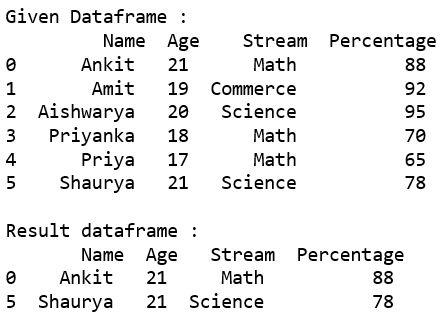My Personal Notes arrow_drop_up